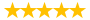# micro exam!!!!!!!!!!

Question # 00005435 Posted By: paul911 Updated on: 12/15/2013 01:42 PM Due on: 12/17/2013
Subject Economics Topic General Economics Tutorials:
Question

. Over a 10-year period, our output growth rate = ?Q/Q = 40%, capital growth rate = ?K/K = 30%, labor growth rate = ?L/L = 20%, the elasticity of output with respect to capital and labor are aK = 0.3 and aL = 0.7 respectively. Find productivity growth. ?Answer:

.

. 13%,

. 11%,

. 17%, or

. 15%

. Question 18

.

3 points

Question 19

. ? Suppose the Fed purchased \$1,200 billion worth of assets in bailing out the financial institutions and in quantitative easing from 2008 to 2011. A. If the deposit reserve requirement ratio is 10%, how much is the potential increase in the U.S. money supply as a result of the Fed’s asset purchase? ?

.

.

. ?B. Suppose that, as a result of the Fed’s loose monetary policy, the private sector’s investment (I) increased by \$700 billion. In addition, as part of the stimulus plan, government spending (G) increased by \$800 billion as well. Given a marginal propensity to consume of 70%, how much would the U.S. GDP increase according to the multiplier model in a closed economy??

.

. ?C. Following the previous question, suppose the marginal propensity to import is 10%, how much would the U.S. GDP increase according to the open-economy multiplier??Your Answer: \$_________billion

.

. ?D. Suppose the Fed’s monetary policy response to the 2008 financial crisis led to an eventual 50% increase in the money supply, how does this affect the aggregate price level in the United States according to the quantity theory of money (assuming the velocity of money and real GDP are constant)??

10 points

Question 20

. ? For a market basket of goods, it costs \$1,000 in the United States, and 6,000 yuan in China (yuan is the unit of the Chinese currency).

.

. A. If the exchange rate is 6 yuan per dollar, where would you buy these goods (assuming there is free trade and no transportation cost between the United States and China)? ?

. Choices are:

. a. Definitely in the United State, not China?

. b. Definitely in China, not the United States

. ?c. Indifferent since the prices in the two countries are the same if they are converted to one or the other currency.?

.

.

. B. Suppose inflation is 4% in the United States and 8.5% in China. What is the new equilibrium exchange rate according to the purchasing power parity??

.

. C. Does the dollar appreciate or depreciate? By how much??

. ?By how much: _________% (keep two digits after the decimal point)??

10 points

Question 21

. ? Refer to the 2010 U.S. GDP information below. (Billions of dollars)

. A. Was the United States total DOMESTIC expenditure (C+I+G) more than, less than, or equal to total output in 2010??

.

. B. How much was the U.S. financial account balance ( IF; in billions of U.S. dollars)??

. Your Answer : \$___________ billion (keep two digits after the decimal point)?

. C. How much was the total domestic saving in the United States ( ST; in billions of U.S. dollars)?

. ?Your Answer: \$___________ billion (keep two digits after the decimal point)?

.

. D. Suppose that the government tax revenue was \$2,000 billion for 2010, what was the U.S. private sector’s saving (SP) in 2010??

. Your Answer: \$_________ billion (keep two digits after the decimal point)?Under gold standard before World War I, the price of one ounce of gold was \$20.67 and £4.2474. Which of the following was an equilibrium exchange rate between the two currencies according to the gold standard?

 a. \$4.8665/£ b. \$0.2055/£ c. £4.8665/\$

Attachments
Tutorials for this Question
1. ## Solution: 100% correct answer

Tutorial # 00005243 Posted By: spqr Posted on: 12/15/2013 01:44 PM
Puchased By: 4
Tutorial Preview
same if they are converted to one or the other ...
Attachments
micro_final_exam_questions_0.doc (370.5 KB)
Recent Feedback
Rated By Feedback Comments Rated On
cel...omdHighly-professional tutors 01/14/2015
new...t33The presentation of work is very effective 01/14/2015

Great! We have found the solution of this question!